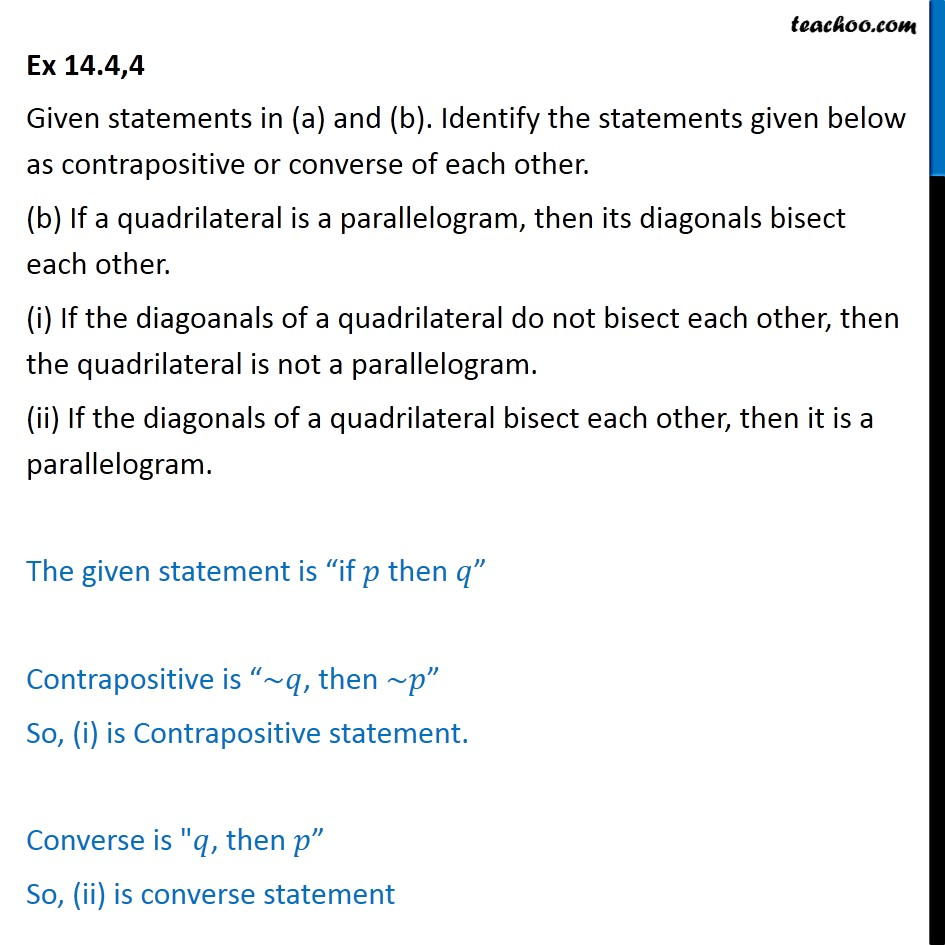If then Statements

Mathematical Reasoning
Serial order wiseLearn in your speed, with individual attention - Teachoo Maths 1-on-1 Class

### Transcript

Question4 Given statements in (a) and (b). Identify the statements given below as contrapositive or converse of each other. (b) If a quadrilateral is a parallelogram, then its diagonals bisect each other. (i) If the diagoanals of a quadrilateral do not bisect each other, then the quadrilateral is not a parallelogram. (ii) If the diagonals of a quadrilateral bisect each other, then it is a parallelogram. The given statement is “if 𝑝 then 𝑞” Contrapositive is “~𝑞, then ~𝑝” So, (i) is Contrapositive statement. Converse is "𝑞, then 𝑝” So, (ii) is converse statement